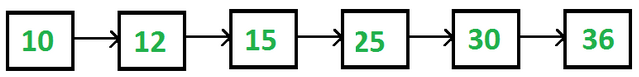61. Consider the following linked list and linked list representation. what will be the value of following statement ?start->next->next->next->data struct node { int data; struct node *next; }*start = NULL;a. 12 b. 30 c. 15 d. 25

 62. If start is pointing to first node of the linked list then consider the following statement -start = start->next;current = start->next;what will be the value of address field of current ? a. 5571 b. 5572 c. 3225 d. 2184

 63. In Linked list implementation, a node carries information regarding _______. a. Link b. Data c. Data and Link d. None of the above

 64. A linked list in which the last node of Linked list points to the first is called a _________. a. Doubly Linked List b. Circular Linked List c. Singly Linked List d. None of these

 65. A doubly linked list performs traversal in _________. a. Any direction b. Circular direction c. Either direction d. None of the above

 66. Linked list data structure usage offers considerable saving in a. Space utilization b. Computational time c. Space utilization & computational time d. None of the above
 Answer: (c).Space utilization & computational time

 67. Consider linked list is used to implement the Stack then which of the following node is considered as Top of the Stack ? a. Any Node b. Last Node c. First Node d. Middle Node